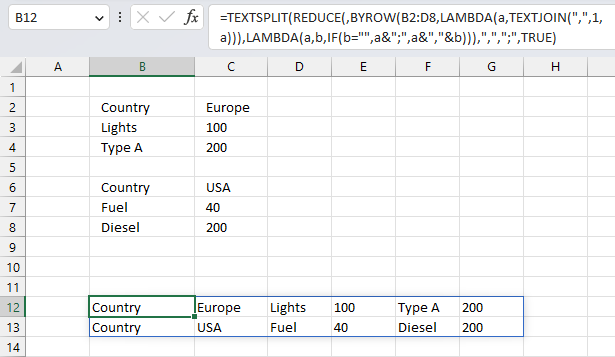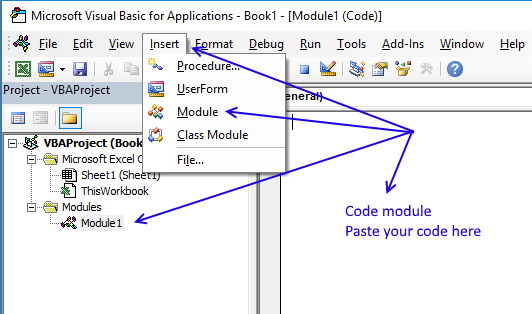Author: Oscar Cronquist Article last updated on March 28, 2023Sheet1A B C D
8 Country Europe
9 Lights 100
10 Type A 200
11
12 Country USA
13 Fuel 40
14 Diesel 200
15
16 Europe Lights Type A 100
17 USA Fuel Diesel 40

Oscar,is there a way to organize this the information into a database format like row 16 onwards,
It picks up all non blanks between the countries putting each line into a separate column.

This article describes two ways to rearrange data to rows based on an empty row as a delimiter. The first one uses the LAMBDA function to rearrange values, the second one demonstrates a User Defined Function that rearranges values.

## 1. Rearrange data - Excel 365 LAMBDA functionThe image above demonstrates a LAMBDA function that rearranges values to single row. An empty row in the source data creates a new row of data in the result.

Excel 365 dynamic array formula:

=TEXTSPLIT(REDUCE(,BYROW(B2:D8,LAMBDA(a,TEXTJOIN(",",1,a))),LAMBDA(a,b,IF(b="",a&";",a&","&b))),",",";",TRUE)

This formula has a limit of 32767 characters, a result larger than that returns an error value.

### Explaining formula

#### Step 1 - Join cell values

The TEXTJOIN function combines text strings from multiple cell ranges.

Function syntax: TEXTJOIN(delimiter, ignore_empty, text1, [text2], ...)

TEXTJOIN(",",1,a)

#### Step 2 - Build LAMBDA function

The LAMBDA function build custom functions without VBA, macros or javascript.

Function syntax: LAMBDA([parameter1, parameter2, …,] calculation)

LAMBDA(a,TEXTJOIN(",",1,a))

#### Step 3 - Perform calculation row by row

The BYROW function puts values from an array into a LAMBDA function row-wise.

Function syntax: BYROW(array, lambda(array, calculation))

BYROW(B2:D8,LAMBDA(a,TEXTJOIN(",",1,a)))

#### Step 4 - Check if value is empty

The IF function returns one value if the logical test is TRUE and another value if the logical test is FALSE.

Function syntax: IF(logical_test, [value_if_true], [value_if_false])

If value is empty a semicolon is appended to the accumulator value, if not a colon is attached.

IF(b="",a&";",a&","&b)

#### Step 5 - Build LAMBDA function

The LAMBDA function build custom functions without VBA, macros or javascript.

Function syntax: LAMBDA([parameter1, parameter2, …,] calculation)

LAMBDA(a,b,IF(b="",a&";",a&","&b))

#### Step 6 - Join values in the array

The REDUCE function shrinks an array to an accumulated value, a LAMBDA function is needed to properly accumulate each value in order to return a total.

Function syntax: REDUCE([initial_value], array, lambda(accumulator, value))

REDUCE(,BYROW(B2:D8,LAMBDA(a,TEXTJOIN(",",1,a))),LAMBDA(a,b,IF(b="",a&";",a&","&b)))

#### Step 7 - Split string based on semicolon and colon

The TEXTSPLIT function splits a string into an array based on delimiting values.

Function syntax: TEXTSPLIT(Input_Text, col_delimiter, [row_delimiter], [Ignore_Empty])

TEXTSPLIT(REDUCE(,BYROW(B2:D8,LAMBDA(a,TEXTJOIN(",",1,a))),LAMBDA(a,b,IF(b="",a&";",a&","&b))),",",";",TRUE)

### Get the Excel fileReorganize-data-Excel-365.xlsx

## 2. Rearrange data - UDFI created a User Defined Function that rearranges non empty cells into rows, using a delimiting value. In the example below, "Country" is the delimiting value. The desired output is displayed in row 11 and 12 and the UDF is shown in row 15 and 16.

A User Defined Function is a custom function that anyone can use, simply copy the VBA code and paste to a code module in your workbook.

Array formula in cell A15:F17

=OrganizeData("Country", A2:C8)

How to enter array formula in cell range A15:F17

• Select cell range A15:F17.
• Type =OrganizeData("Country", A2:C8)
• Press and hold CTRL + SHIFT simultaneously.
• Press Enter once.
• Release all keys.

### User defined Function Syntax

OrganizeData(srch, rng)

### Arguments

 srch Required. A delimiting value. rng Required. The range containing values you want to rearrange.

### VBA code

```'Name User Defined Function
Function OrganizeData(srch As String, rng As Variant)

'Declare variables and data types
Dim cell As Range, temp() As Variant, ca As Single
Dim iRows As Integer, i As Integer, c As Single, r As Single
Dim chk As Boolean

'Make array temp as large as the cell range you entered the UDF in

'Save False to variable chk
chk = False

'Save values in cell range rng to array variable rng
rng = rng.Value

'Iterate through rows in rng variable
For r = LBound(rng, 1) To UBound(rng, 1)

'Iterate through columns in array variable
For c = LBound(rng, 2) To UBound(rng, 2)

'If rng value is equal to delimiting value
If rng(r, c) = srch Then

'If Chk variable is not equal to False
If chk <> False Then

'Save blanks to temp variable based on value i
For ca = i To UBound(temp, 1)
temp(ca, UBound(temp, 2)) = ""
Next ca

'Reset i to 0 (zero)
i = 0

'Increase array variable temp by 1
ReDim Preserve temp(UBound(temp, 1), UBound(temp, 2) + 1)
End If

'Save True to variable chk
chk = True

'If rng variable is not equal to nothing and rng variable is not equal to delimiting value then
ElseIf rng(r, c) <> "" And rng(r, c) <> srch Then

'Save value to array variable temp
temp(i, UBound(temp, 2)) = rng(r, c)

'Increment i with 1
i = i + 1
End If
Next c
Next r
'Save blanks to remaining values in array variable temp
For ca = i To UBound(temp, 1)
temp(ca, UBound(temp, 2)) = ""
Next ca

'Increase containers in arrat variable temp with 1
ReDim Preserve temp(UBound(temp, 1), UBound(temp, 2) + 1)

'Count the number of rows you have entered the UDF in

'Save blanks to remaining cells
For r = UBound(temp, 2) To iRows
For c = LBound(temp, 1) To UBound(temp, 1)
temp(c, r) = ""
Next c
ReDim Preserve temp(UBound(temp, 1), UBound(temp, 2) + 1)
Next r

'Return values in temp to worksheet rearranged vertically
OrganizeData = Application.Transpose(temp)

End Function
```

### Where to copy the code?

1. Copy VBA code above.
2. Press Alt+ F11 to open the Visual Basic Editor.3. Press with left mouse button on "Insert" on the top menu.
4. Press with left mouse button on "Module" to create a module.
5. Paste code to module